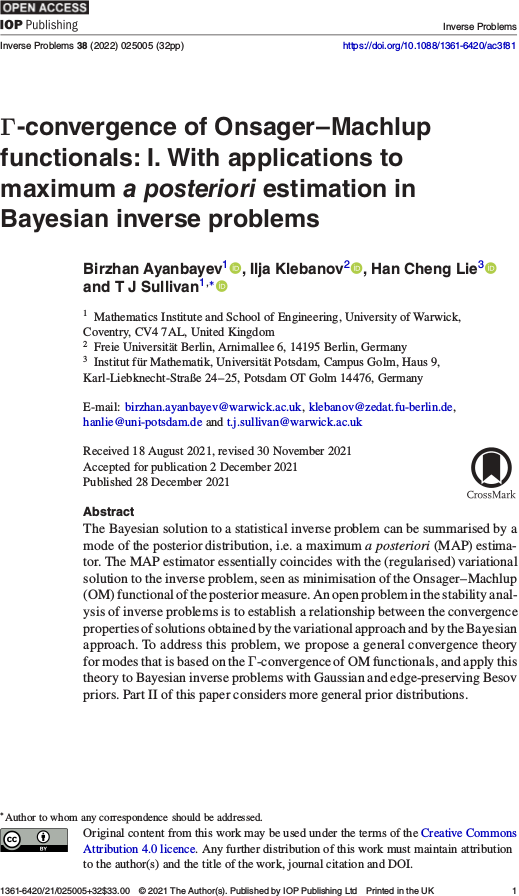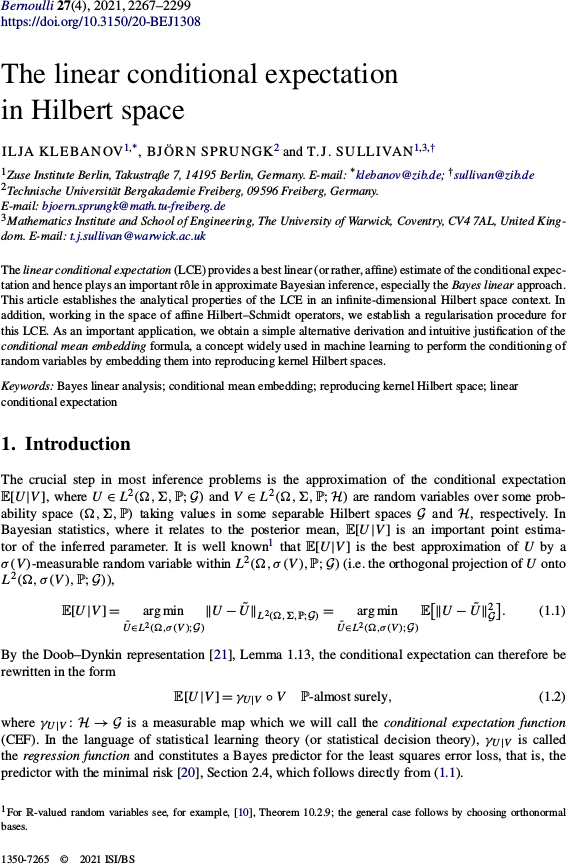# Tim Sullivan

### #publication

Clear Search### Randomised integration for deterministic operator differential equations in Calcolo

The article “Randomised one-step time integration methods for deterministic operator differential equations” by Han Cheng Lie, Martin Stahn, and myself has just appeared in its final form in Calcolo. In this paper, we extend the analysis of Conrad et al. (2016) and Lie et al. (2019) to the case of evolutionary systems in Banach spaces or even Gel′fand triples, this being the right setting for many evolutionary partial differential equations.

H. C. Lie, M. Stahn, and T. J. Sullivan. “Randomised one-step time integration methods for deterministic operator differential equations.” Calcolo 59(1):13, 33pp., 2022. doi:10.1007/s10092-022-00457-6

Abstract. Uncertainty quantification plays an important role in applications that involve simulating ensembles of trajectories of dynamical systems. Conrad et al. (Stat. Comput., 2017) proposed randomisation of deterministic time integration methods as a strategy for quantifying uncertainty due to time discretisation. We consider this strategy for systems that are described by deterministic, possibly non-autonomous operator differential equations defined on a Banach space or a Gel′fand triple. We prove pathwise and expected error bounds on the random trajectories, given an assumption on the local truncation error of the underlying deterministic time integration and an assumption that the absolute moments of the random variables decay with the time step. Our analysis shows that the error analysis for differential equations in finite-dimensional Euclidean space carries over to infinite-dimensional settings.

Published on Friday 25 February 2022 at 17:00 UTC #publication #prob-num #lie #stahn### Γ-convergence of Onsager-Machlup functionals in Inverse Problems

The articles “Γ-convergence of Onsager–Machlup functionals” (“I. With applications to maximum a posteriori estimation in Bayesian inverse problems” and “II. Infinite product measures on Banach spaces”) by Birzhan Ayanbayev, Ilja Klebanov, Han Cheng Lie, and myself have just appeared in their final form in the journal Inverse Problems.

The purpose of this work is to address a long-standing issue in the Bayesian approach to inverse problems, namely the joint stability of a Bayesian posterior and its modes (MAP estimators) when the prior, likelihood, and data are perturbed or approximated. We show that the correct way to approach this problem is to interpret MAP estimators as global weak modes in the sense of Helin and Burger (2015), which can be identified as the global minimisers of the Onsager–Machlup functional of the posterior distribution, and hence to provide a convergence theory for MAP estimators in terms of Γ-convergence of these Onsager–Machlup functionals. It turns out that posterior Γ-convergence can be assessed in a relatively straightforward manner in terms of prior Γ-convergence and continuous convergence of potentials (negative log-likelihoods). Over the two parts of the paper, we carry out this programme both in generality and for specific priors that are commonly used in Bayesian inverse problems, namely Gaussian and Besov priors (Lassas et al., 2009; Dashti et al., 2012).

B. Ayanbayev, I. Klebanov, H. C. Lie, and T. J. Sullivan. “Γ-convergence of Onsager–Machlup functionals: I. With applications to maximum a posteriori estimation in Bayesian inverse problems.” Inverse Problems 38(2):025005, 32pp., 2022. doi:10.1088/1361-6420/ac3f81

Abstract (Part I). The Bayesian solution to a statistical inverse problem can be summarised by a mode of the posterior distribution, i.e. a MAP estimator. The MAP estimator essentially coincides with the (regularised) variational solution to the inverse problem, seen as minimisation of the Onsager–Machlup functional of the posterior measure. An open problem in the stability analysis of inverse problems is to establish a relationship between the convergence properties of solutions obtained by the variational approach and by the Bayesian approach. To address this problem, we propose a general convergence theory for modes that is based on the Γ-convergence of Onsager–Machlup functionals, and apply this theory to Bayesian inverse problems with Gaussian and edge-preserving Besov priors. Part II of this paper considers more general prior distributions.

B. Ayanbayev, I. Klebanov, H. C. Lie, and T. J. Sullivan. “Γ-convergence of Onsager–Machlup functionals: II. Infinite product measures on Banach spaces.” Inverse Problems 38(2):025006, 35pp., 2022. doi:10.1088/1361-6420/ac3f82

Abstract (Part II). We derive Onsager–Machlup functionals for countable product measures on weighted $$\ell^{p}$$ subspaces of the sequence space $$\mathbb{R}^\mathbb{N}$$. Each measure in the product is a shifted and scaled copy of a reference probability measure on $$\mathbb{R}$$ that admits a sufficiently regular Lebesgue density. We study the equicoercivity and Γ-convergence of sequences of Onsager–Machlup functionals associated to convergent sequences of measures within this class. We use these results to establish analogous results for probability measures on separable Banach or Hilbert spaces, including Gaussian, Cauchy, and Besov measures with summability parameter $$1 \leq p \leq 2$$. Together with Part I of this paper, this provides a basis for analysis of the convergence of maximum a posteriori estimators in Bayesian inverse problems and most likely paths in transition path theory.

Published on Wednesday 5 January 2022 at 12:00 UTC #publication #inverse-problems #modes #map-estimators #ayanbayev #klebanov #lie### Linear conditional expectation in Hilbert space in Bernoulli

The article “The linear conditional expectation in Hilbert space” by Ilja Klebanov, Björn Sprungk, and myself has just appeared in its final form in the journal Bernoulli. In this paper, we study the best approximation $$\mathbb{E}^{\mathrm{A}}[U|V]$$ of the conditional expectation $$\mathbb{E}[U|V]$$ of an $$\mathcal{G}$$-valued random variable $$U$$ conditional upon a $$\mathcal{H}$$-valued random variable $$V$$, where “best” means $$L^{2}$$-optimality within the class $$\mathrm{A}(\mathcal{H}; \mathcal{G})$$ of affine functions of the conditioning variable $$V$$. This approximation is a powerful one and lies at the heart of the Bayes linear approach to statistical inference, but its analytical properties, especially for $$U$$ and $$V$$ taking values in infinite-dimensional spaces $$\mathcal{G}$$ and $$\mathcal{H}$$, are only partially understood — which this article aims to rectify.

I. Klebanov, B. Sprungk, and T. J. Sullivan. “The linear conditional expectation in Hilbert space.” Bernoulli 27(4):2267–2299, 2021. doi:10.3150/20-BEJ1308

Abstract. The linear conditional expectation (LCE) provides a best linear (or rather, affine) estimate of the conditional expectation and hence plays an important rôle in approximate Bayesian inference, especially the Bayes linear approach. This article establishes the analytical properties of the LCE in an infinite-dimensional Hilbert space context. In addition, working in the space of affine Hilbert–Schmidt operators, we establish a regularisation procedure for this LCE. As an important application, we obtain a simple alternative derivation and intuitive justification of the conditional mean embedding formula, a concept widely used in machine learning to perform the conditioning of random variables by embedding them into reproducing kernel Hilbert spaces.

Published on Wednesday 25 August 2021 at 08:00 UTC #publication #tru2 #bayesian #rkhs #mean-embedding #klebanov #sprungk### Computing with dense kernel matrices at near-linear cost in MMS

The paper “Compression, inversion, and approximate PCA of dense kernel matrices at near-linear computational complexity” by Florian Schäfer, Houman Owhadi, and myself has just appeared in print in Multiscale Modeling and Simulation. This paper shows how a surprisingly simple algorithm — the zero fill-in incomplete Cholesky factorisation — with respect to a cleverly-chosen sparsity pattern allows for near-linear complexity compression, inversion, and approximate PCA of square matrices of the form

$$\Theta = \begin{bmatrix} G(x_{1}, x_{1}) & \cdots & G(x_{1}, x_{N}) \\ \vdots & \ddots & \vdots \\ G(x_{N}, x_{1}) & \cdots & G(x_{N}, x_{N}) \end{bmatrix} \in \mathbb{R}^{N \times N} ,$$

where $$\{ x_{1}, \dots, x_{N} \} \subset \mathbb{R}^{d}$$ is a data set and $$G \colon \mathbb{R}^{d} \times \mathbb{R}^{d} \to \mathbb{R}$$ is a covariance kernel function. Such matrices play a key role in, for example, Gaussian process regression and RKHS-based machine learning techniques.

F. Schäfer, T. J. Sullivan, and H. Owhadi. “Compression, inversion, and approximate PCA of dense kernel matrices at near-linear computational complexity.” Multiscale Modeling & Simulation: A SIAM Interdisciplinary Journal 19(2):688–730, 2021. doi:10.1137/19M129526X

Abstract. Dense kernel matrices $$\Theta \in \mathbb{R}^{N \times N}$$ obtained from point evaluations of a covariance function $$G$$ at locations $$\{ x_{i} \}_{1 \leq i \leq N}$$ arise in statistics, machine learning, and numerical analysis. For covariance functions that are Green's functions of elliptic boundary value problems and homogeneously-distributed sampling points, we show how to identify a subset $$S \subset \{ 1 , \dots , N \}^2$$, with $$\# S = O ( N \log (N) \log^{d} ( N /\varepsilon ) )$$, such that the zero fill-in incomplete Cholesky factorisation of the sparse matrix $$\Theta_{ij} 1_{( i, j ) \in S}$$ is an $$\varepsilon$$-approximation of $$\Theta$$. This factorisation can provably be obtained in complexity $$O ( N \log( N ) \log^{d}( N /\varepsilon) )$$ in space and $$O ( N \log^{2}( N ) \log^{2d}( N /\varepsilon) )$$ in time; we further present numerical evidence that $$d$$ can be taken to be the intrinsic dimension of the data set rather than that of the ambient space. The algorithm only needs to know the spatial configuration of the $$x_{i}$$ and does not require an analytic representation of $$G$$. Furthermore, this factorization straightforwardly provides an approximate sparse PCA with optimal rate of convergence in the operator norm. Hence, by using only subsampling and the incomplete Cholesky factorization, we obtain, at nearly linear complexity, the compression, inversion and approximate PCA of a large class of covariance matrices. By inverting the order of the Cholesky factorization we also obtain a solver for elliptic PDE with complexity $$O ( N \log^{d}( N /\varepsilon) )$$ in space and $$O ( N \log^{2d}( N /\varepsilon) )$$ in time.

Published on Thursday 15 April 2021 at 12:00 UTC #publication #prob-num #schaefer #owhadi### Convergence rates of Gaussian ODE filters in Statistics and Computing

The paper “Convergence rates of Gaussian ODE filters” by Hans Kersting, Philipp Hennig, and myself has just appeared in the journal Statistics and Computing. In this work, we examine the strong convergence rates of probabilistic solvers for ODEs of the form $$\dot{x}(t) = f(x(t))$$ that are based upon Gaussian filtering. In some sense, this work combines the numerical analysis perspective of Conrad et al. (2016) and Lie et al. (2019) with the filtering perspective on probabilistic numerical methods for ODEs of Schober et al. (2014).

H. Kersting, T. J. Sullivan, and P. Hennig. “Convergence rates of Gaussian ODE filters.” Statistics and Computing 30(6):1791–1816, 2020. doi:10.1007/s11222-020-09972-4

Abstract. A recently introduced class of probabilistic (uncertainty-aware) solvers for ordinary differential equations (ODEs) applies Gaussian (Kalman) filtering to initial value problems. These methods model the true solution $$x$$ and its first $$q$$ derivatives a priori as a Gauss–Markov process $$X$$, which is then iteratively conditioned on information about $$\dot{x}$$. This article establishes worst-case local convergence rates of order $$q + 1$$ for a wide range of versions of this Gaussian ODE filter, as well as global convergence rates of order $$q$$ in the case of $$q = 1$$ and an integrated Brownian motion prior, and analyses how inaccurate information on $$\dot{x}$$ coming from approximate evaluations of $$f$$ affects these rates. Moreover, we show that, in the globally convergent case, the posterior credible intervals are well calibrated in the sense that they globally contract at the same rate as the truncation error. We illustrate these theoretical results by numerical experiments which might indicate their generalizability to $$q \in \{ 2, 3 , \dots \}$$.

Published on Tuesday 15 September 2020 at 09:00 UTC #publication #stco #prob-num #kersting #hennig